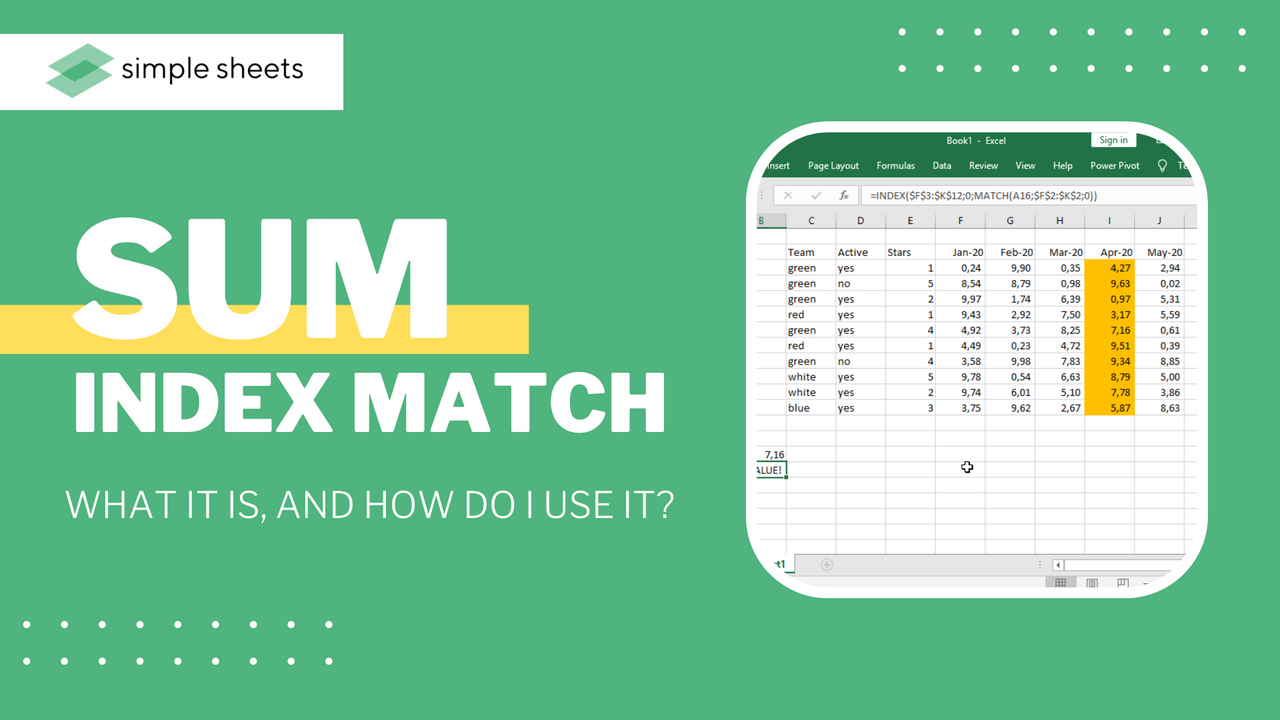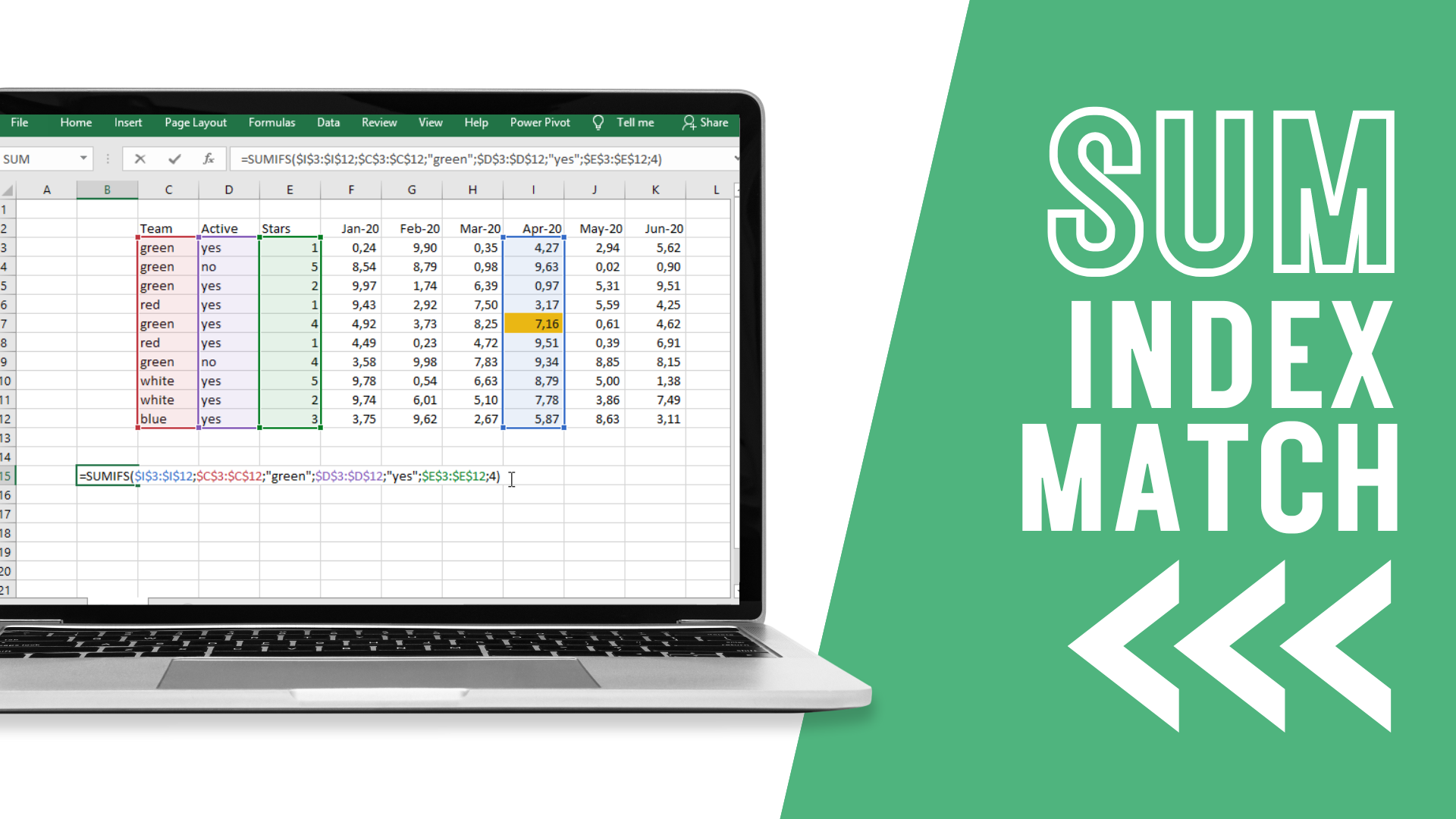# SUM Index-Match: What it is, and How do I use it?

Jul 26, 2022When you find yourself handling large quantities of data within Excel, it is common to implement the INDEX and MATCH functions to search parameters under more than one criteria for sum.

This article will teach you how to incorporate SUM, SUMIFS, and SUMPRODUCT alongside the INDEX-MATCH formula to sum under several criteria in Excel. You can also watch the video below if you are a visual learner.

## Introduction to the Functions: SUM, INDEX, and MATCH with Examples

### SUM

The objective of the SUM function is to sum all the numbers from a range of chosen cells. The formula syntax looks like this: =SUM(number1, [number2],…)

### INDEX

The objective of the INDEX function is to return a value of reference for the cell that intersects a chosen column and row in a selected range. Here is the formula syntax: =INDEX(array, row_num, [column_num]) or, =INDEX(reference, row_num, [column_num], [area_num])

### MATCH

The objective of the MATCH function is to return the relative position of a chosen item in an array that mirrors a specific value in a specific order. The formula syntax is as follows: =MATCH(lookup_value, lookup_array, [match_type])## Use of INDEX and MATCH Functions Together in Excel

Now you understand how to use INDEX and MATCH in Excel; you can use the INDEX and MATCH functions together. The INDEX and MATCH function is excellent for finding specific data from a big array.

In this function, MATCH focuses on the row & column positions of the input values. In contrast, the INDEX function focuses on the output from the intersection of that column and row position.

## Use of SUMIF with INDEX-MATCH Functions to Sum under Multiple Criteria

Before we move on to another type of combined formula, let's look at the SUMIF function. This one of the sum functions aims to add the cells specified by chosen criteria or conditions. =SUMIF(range, criteria, [sum_range])

## Use of SUMIFS with INDEX & MATCH Functions in Excel

SUMIFS is a sub-formula of the SUMIF formula. If you use the SUMIFS function with the INDEX and MATCH functions inside, you can add more than one criterion, which you can't do by just using the SUMIF function. To do this, ensure you input your Sum Range, then Criteria Range, then Range Criteria.## Concluding Words

Now that you understand the methods we discussed in this article, it's time to apply this information to your next Excel project. Keep an eye on the Excel index function returns to ensure they match your expectations, and you'll quickly retrieve individual values faster and easier!### How do I sum multiple cells with an INDEX match?

There are three easy ways to sum multiple cells with the INDEX match function:

1. SUM family function
2. AGGREGATE function
3. SUBTOTAL function

### How does a sum INDEX work?

The SUM function in Excel adds every number in a chosen range of cells and provides the sum of those values. The INDEX function offers the value at a chosen index in an array.## Related Articles:

Free Microsoft Excel Tutorial For Beginners

#### Want to Make Excel Work for You? Try out 5 Amazing Excel Templates & 5 Unique Lessons

We hate SPAM. We will never sell your information, for any reason.